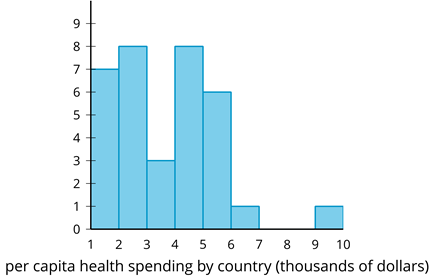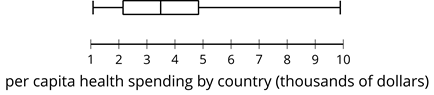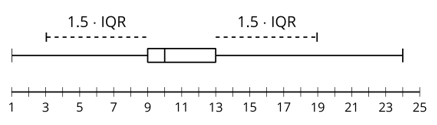# A.1.14.1 Health Care Spending

The histogram and box plot show the average amount of money, in thousands of dollars, spent on each person in the country (per capita spending) for health care in 34 countries.One value in the set is an outlier. Which one is it? What is its approximate value?

By one rule for deciding (this is what we will use), a value is an outlier if it is more than 1.5 times the IQR greater than Q3 or less than Q1 -- in other words: (IQR*1.5 + Q3) or (Q1 - IQR*1.5). Use one of these formulas to decide whether or not your value from above meets this definition of an outlier.

Here is a picture of the definition of an outlier (for a different example). The dashed lines represent the legnth added or subtracted to the quartiles. This set has outliers on each end (large and small).